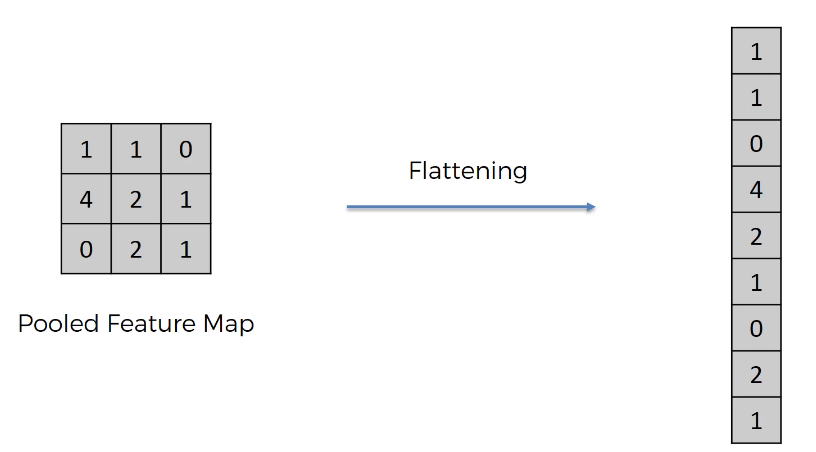# The Flattening and Full Connection Steps of Convolutional Neural Networks

Hey - Nick here! This page is a free excerpt from my \$199 course Python for Finance, which is 50% off for the next 50 students.

So far in our discussion of convolutional neural networks, you have learned:

In this tutorial, you will learn about the next two steps in building a convolutional neural network: the flattening and full connection steps.

## The Flattening Step in Convolutional Neural Networks

The flattening step is a refreshingly simple step involved in building a convolutional neural network.

It involves taking the `pooled feature map` that is generated in the pooling step and transforming it into a one-dimensional vector. Here is a visual representation of what this process looks like:The reason why we transform the `pooled feature map` into a one-dimensional vector is because this vector will now be fed into an artificial neural network. Said differently, this vector will now become the input layer of an artificial neural network that will be chained onto the convolutional neural network we've been building so far in this course.

## The Full Connection Step in Convolutional Neural Networks

As you can likely infer from the last section, the full connection step involves chaining an artificial neural network onto our existing convolutional neural network.

The reason this is called the full connection step is because the hidden layer of the artificial neural network is replaced by a specific type of hidden layer called a `fully connected layer`. As its name implies, a `fully connected` layer's neurons are connected to all of the neurons in the next layer.

Here is a visual example of a fully connected layer in an artificial neural network:The purpose of the fully connected layer in a convolutional neural network is to detect certain features in an image. More specifically, each neuron in the fully connected layer corresponds to a specific feature that might be present in an image. The value that the neuron passes on to the next layer represents the probability that the feature is contained in the image.

The end of the artificial neural network coincides with the end of the convolutional neural network. Said differently, the artificial neural network at the end of a CNN predicts what's contained in the image that the CNN is attempting to recognize!

## Final Thoughts

In this tutorial, you had a brief, no-code introduction to the flattening and full connection steps within convolutional neural networks.

Here is a brief summary of what you learned:

• How the flattening step transforms the `feature map` into a one-dimensional matrix that is used as the input layer in an artificial neural network appended to the end of the CNN
• That the fully connected step involves building an ANN where each hidden layer is a fully connected layer
• The ANN at the end of the CNN actually calculates the prediction for the overall CNN